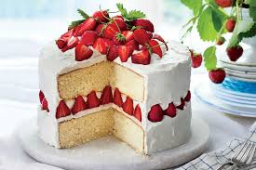# Three cakes

There are three cakes an ice cream cake, chocolate, and a sponge cake. We ate 3/4 of the ice cream cake. We cut the chocolate cake into twelve equal pieces, of which We ate nine. The sponge cake was divided into eight equal pieces, with only one remaining.

a) What fraction of each cake was eaten?
b) What fraction of each cake was left over?
c) What is the total amount of cake eaten during the party?
d) What is the total amount of cake left over after the party?

a1 =  3/4
a2 =  3/4
a3 =  7/8
b1 =  1/4
b2 =  1/4
b3 =  1/8
c =  19/8 = 2 38
d =  5/8

### Step-by-step explanation:Did you find an error or inaccuracy? Feel free to write us. Thank you!

Tips for related online calculators
Need help calculating sum, simplifying, or multiplying fractions? Try our fraction calculator.